## Electrochemistry

Our Electrochemical Workstations ZENNIUM X / ZENNIUM Pro / ZENNIUM  base on an universal and modular data acquisition system. Together with the THALES software package we offer all standard electrochemical methods at a mouse click.## Photo-Electrochemistry

CIMPS is an universal photo- and spectro-electrochemical workstation for a wide field of applications. You can easily extend the basic CIMPS system with various options for special fields of photo-electrochemical research.## Energy Storage & Conversion

Our power potentiostats, electronic loads and multi-channels acquisition systems provide a smart system for research and investigation on batteries, fuel cells and super caps.## Corrosion

Electrochemical noise has become popular as a new method of corrosion detection and prevention. ZAHNER has developed the CorrElNoise® technique, the first method to obtain both, potential noise and current noise from one electrochemical source.## The Normalization of the CPE

Usually, modern potentiostats and galvanostats involve a couple of electrochemical investigation methods which are of big relevance for the improvement of existing- and the development of new products. Electrochemical Impedance Spectroscopy (EIS) is one of the most important techniques for the determination of the major parameters of these products, if this method is implemented in the electrochemical workstation. Fuel cells, batteries and/or rechargeable batteries, supercaps, dielectrics as well as coatings for corrosion prevention are the main areas of interest. Subsequently in these systems, “energy storing components” like capacities are found in the recorded impedance spectra. For the theoretical explanation of these spectra, the impedance (ZC) of these components is often described using an ideal capacitor (C – see eq. 1).

Unfortunately, most often the “theoretical capacitors C” do not model the practical spectra of these technical systems adequately. Since the difference between the theoretical description and the real findings in these systems is found systematically, a non ideal component characterizing a non ideal capacity has been introduced decades ago. This “energy storing component” which possesses the physical meaning of a loss capacity, is denoted as Constant Phase Elements (CPE - Y0, see eq. 1) and is used commonly in the description/interpretation of the impedance (ZCPE) in the corresponding electrochemical impedance spectra.

However, the unit of a CPE (Y0) depends on the value of the exponent α (eq. 1). To make different CPE-Elements comparable, the CPE has to be normalized. This can be done, setting the impedance of the CPE (ZCPE) as a function of the angular frequency ω (=2π·f) equal to the impedance of an ideal capacitor (ZC). The corresponding expressions for the impedances are summarized in eq. 1.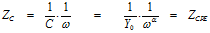(eq. 1)

Rearrangement of equation eq. 1 yields eq. 2 as an expression for the evaluation of an equivalent capacity from a CPE Y0. One immediately recognizes that the correspondence of the impedances of CPE and C only is valid only at a particular frequency. This frequency is called the normalizing frequency ωnorm (see eq. 2, right hand part).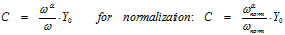(eq. 2)

All one has to do is to select an appropriate normalizing frequency (f) which has to be chosen carefully. As one can see immediately from figure 1, the value of the equivalent capacity is a function of the (normalizing) frequency. Without explicit normalization, an “invisible” implicit normalization at 1/2pi; is in effect. This may be cumbersome since often, the impedance of a capacity is not the dominating impedance at this quite low frequency. Neglecting this fact may result in huge errors interpreting a CPE in terms of a capacity.

As a rule of thumb, dominating capacitive behavior is often observed in a higher frequency range, setting 1000 Hz as an intuitive approximation for a standard normalization frequency.

Concerning the definition given in eq. 1, one has to conclude that the CPE must be the dominant element at this frequency in the impedance spectrum at the chosen frequency (f). Otherwise the impedance at this frequency cannot be chosen as normalizing frequency as long as the impedance is dominated by another element.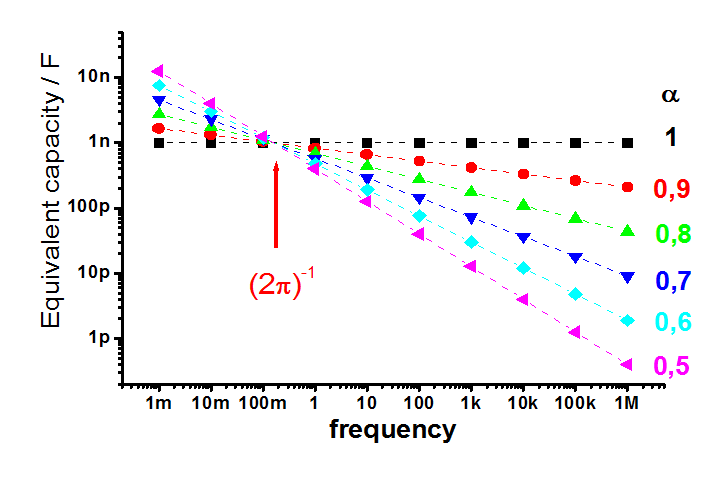Figure 1: Calculated equivalent capacity of a CPE-element Y0 (value of (1 nF) π ) as a function of the frequency for different exponents α in logarithmic scale

Concerning the special case where the CPE-element is in parallel to a resistor, it is possible to evaluate the equivalent capacity in an unambiguous way. According to equation eq. 3, one can define the impedance of the parallel combination of a resistor and a capacitive-type element in different ways (see also table 1).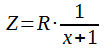(eq. 3)

 Element combination Simple RC-element ”Cole-Cole“-type CPE (Y0)-type Expression for x RC·(jω) (λ·jω)α with λ = R·C λ‘·(jω)α with λ‘ = R·Y0

Table 1: Definition of combinations of a resistor and a capacitive-type element according to equation eq. 3

Therefore, combining the “Cole-Cole”-type impedance with that of the parallel combination of the CPE Y0 and the resistor R, one obtains an expression for the equivalent capacity which is independent of the (angular) frequency.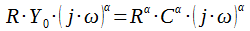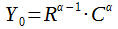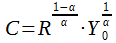Summary 1: Evaluating an equivalent capacity of a CPE in a parallel combination of a CPE Y0 and a resistor R obtained by comparing the impedance with the “Cole-Cole”-type transfer function

This formula is sometimes called the DeBrug-formula and enables the evaluation of a capacity from a combination of an R with a CPE-element. Besides a parallel-arrangement of these two elements, a capacity of a Randle’s arrangement may result in the same formula. For details, please refer to the paper of Hirschorn which is given at the end of this section.

## References

K.S. Cole, R.H. Cole;J. Chem. Phys. 9 (1941) 341–352

K.S. Cole, R.H. Cole; J. Chem. Phys. 10 (1942) 98-105

G. J. Brug, A. L. G. van den Eeden, M. Sluyters-Rehbach, J. H. Sluyters; Journal of Electroanalytic Chemistry 176 (1984) 275-295

C.H. Hsu, F. Mansfeld; Corrosion 57 / No. 9 (2001) 747-748

M.R. Shoar Abouzari, F. Berkemeier, G. Schmitz, D. Wilmer; Solid State Ionics 180 (2009) 922–927

B. Hirschorn, M. Orazem, B. Tribollet, V. Vivier, I. Frateur, M. Musiani; El. Acta 55 (2010) 6218–6227

## Software tool

To facilitate the normalization of the CPE according to equation eq. 1 or summary 1 respectively, a small software tool was implemented. The tool (CPE_Normalization.exe) can be copied into any folder one desires; there is no need of any entries in registry or initialization file and the execution is predominantly self-explanatory.

The first three entries (see figure 2), i.e. “CPE-element Y0 [F^a]”, “Exponent (a)” and “Normalize at [Hz]” correspond to exactly the same elements described above. The entry “Resistor [Ohm]” enables the differentiation between the use of the normalizing formula(s) according to equation eq.2 and summary 1.

• Inserting a value (>10-8 Ω) for the resistance will evaluate the equivalent capacity in a parallel combination of a CPE Y0 and a resistor according to summary 1.

• Selecting “0=none” (by open up the input box) or inserting a non-valid numerical expression (<10-8 Ω or a negative value) will calculate the normalized equivalent capacity of the CPE Y0 according to equation eq. 2.

In the latter case, the entry “Old normalization at [Hz]” can be used to re-evaluate the capacity of a CPE-element Y0 which has already been normalized at another frequency. For instance, in the Zahner software package the normalization frequency is set to 1000 Hz (i.e. ωnorm.=2π·1000 Hz).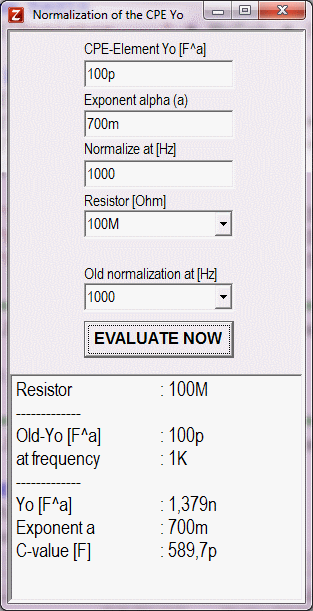Figure 2: Software tool for the normalization of the CPE Y0

The result of the evaluation procedure as well as the relevant entries is summarized in the text-field below the “EVALUATE NOW” button which can be copied into the clipboard for further use.